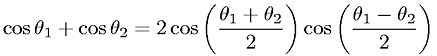Equations > Trigonometry > Trigonometric Identities > Superposition (Addition) of Cosine of Angles

Superposition (Addition) of Cosine of AnglesLatex Code:

MathML Code:

 $\mathrm{cos}{\theta }_{1}+\mathrm{cos}{\theta }_{2}=2\mathrm{cos}\left(\frac{\theta }_{1}+{\theta }_{2}2\right)\mathrm{cos}\left(\frac{\theta }_{1}-{\theta }_{2}2\right)$

MathType 5.0: